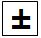Succeed with maths – Part 1

Start this free course now. Just create an account and sign in. Enrol and complete the course for a free statement of participation or digital badge if available.

Free course

# 6 Calculator exploration: negative numbers

You will now learn how to use a calculator for negative numbers – something that can make life a lot easier!

You have plenty of options when it comes to what calculator to use and you may find it easier to use the one that you are most familiar with. This could be a calculator on your mobile phone, on your computer or tablet, an online calculator, or a handheld calculator.

To enter a negative number on a calculator you will usually use the(plus/minus) key.

Find this key now.

How you enter a negative number will depend upon the calculator you are using. For most newer calculators you will need to press thekey and then enter the number.

Some may require you to enter the number and then press the key.

You may be wondering which key to use on your keyboard for negative numbers. There is no direct equivalent of thebutton, but the minus key performs the same task.

## Table _unit8.6.1 Table 1 Minus operator buttons

Mathematical operation Calculator button Keyboard key
Negative numberThe − (minus) key

Now you can have a go at some examples using a calculator.

## Activity _unit8.6.1 Activity 7 Adding and subtracting negative numbers using the calculator

Timing: Allow approximately 10 minutes

Work out the answers to the following questions in your head first to get some more practice. Then check that they are correct on the calculator.

• a.2 + (–5)

• a.Remember the rule that adding a negative number is the same as subtracting the corresponding positive number.

2 + (–5) = 2 − 5 = –3

• b.(–4) − 3

• b.(–4) − 3 = –7
• c.8 − (–5)

Remember the rule that subtracting a negative number is the same as adding the corresponding positive number.

• c.8 − (–5) = 8 + 5 = 13
• d.(–10) + (–2)

• d.(–10) + (–2) = (–10) − 2 = –12
• e.(–3) + 3

• e.(–3) + 3 = 0